Main Content

# Reconstruction Through Two-Channel Filter Bank

This example shows how to design perfect reconstruction two-channel filter banks, also known as the Quadrature Mirror Filter (QMF) Banks since they use power complementary filters.

Often in digital signal processing the need arises to decompose signals into low and high frequency bands, after which need to be combined to reconstruct the original signal. Such an example is found in subband coding (SBC).

This example will first simulate the perfect reconstruction process by filtering a signal made up of Kronecker deltas. Plots of the input, output, and error signal are provided, as well as the magnitude spectrum of transfer function of the complete system. The effectiveness of the perfect reconstruction is shown through this filter bank. After that, an example application shows how the two subbands of an audio file can be processed differently without much effect on the reconstruction.

### Perfect Reconstruction

Perfect reconstruction is a process by which a signal is completely recovered after being separated into its low frequencies and high frequencies. Below is a block diagram of a perfect reconstruction process which uses ideal filters. The perfect reconstruction process requires four filters, two lowpass filters (H0 and G0) and two highpass filters (H1 and G1). In addition, it requires a downsampler and upsampler between the two lowpass and between the two highpass filters. Note that we have to account for the fact that our output filters need to have a gain of two to compensate for the preceding upsampler.### Perfect Reconstruction Two-Channel Filter Bank

The DSP System Toolbox™ provides a specialized function, called FIRPR2CHFB, to design the four filters required to implement an FIR perfect reconstruction two-channel filter bank as described above. FIRPR2CHFB designs the four FIR filters for the analysis (H0 and H1) and synthesis (G0 and G1) sections of a two-channel perfect reconstruction filter bank. The design corresponds to so-called orthogonal filter banks also known as power-symmetric filter banks, which are required in order to achieve the perfect reconstruction.

Let's design a filter bank with filters of order 99 and passband edges of the lowpass and highpass filters of 0.45 and 0.55, respectively:

```N = 99; [LPAnalysis, HPAnalysis, LPSynthsis, HPSynthesis] = firpr2chfb(N, 0.45); ```

The magnitude response of these filters is plotted below:

```fvt = fvtool(LPAnalysis,1, HPAnalysis,1, LPSynthsis,1, HPSynthesis,1); fvt.Color = [1,1,1]; legend(fvt,'Hlp Lowpass Decimator','Hhp Highpass Decimator',... 'Glp Lowpass Interpolator','Ghp Highpass Interpolator'); ```Note that the analysis path consists of a filter followed by a downsampler, which is a decimator, and the synthesis path consists of an upsampler followed by a filter, which is an interpolator. The DSP System Toolbox™ provides two System objects to implement this - `dsp.SubbandAnalysisFilter` for analysis and `dsp.SubbandSynthesisFilter` for the synthesis section.

```analysisFilter = dsp.SubbandAnalysisFilter(LPAnalysis, HPAnalysis); % Analysis section synthFilter = dsp.SubbandSynthesisFilter(LPSynthsis, HPSynthesis); % Synthesis section ```

For the sake of an example, let p[n] denote the signaland let the signal x[n] be defined byNOTE: Since MATLAB® uses one-based indexing, delta[n]=1 when n=1.

```x = zeros(50,1); x(1:3) = 1; x(8:10) = 2; x(16:18) = 3; x(24:26) = 4; x(32:34) = 3; x(40:42) = 2; x(48:50) = 1; sigsource = dsp.SignalSource('SignalEndAction', 'Cyclic repetition',... 'SamplesPerFrame', 50); sigsource.Signal = x; ```

To view the results of the simulation, we will need three scopes - first to compare the input signal with the reconstructed output, second to measure the error between the two and third to plot the magnitude response of the overall system.

```% Scope to compare input signal with reconstructed output sigcompare = dsp.ArrayPlot('NumInputPorts', 2, 'ShowLegend', true,... 'Title', 'Input (channel 1) and reconstructed (channel 2) signals'); % Scope to plot the RMS error between the input and reconstructed signals errorPlot = timescope('Title', 'RMS Error', 'SampleRate', 1, ... 'TimeUnits', 'Seconds', 'YLimits', [-0.5 2],... 'TimeSpanSource', 'property','TimeSpan',100,... 'TimeSpanOverrunAction','Scroll'); % To calculate the transfer function of the cascade of Analysis and % Synthesis subband filters tfestimate = dsp.TransferFunctionEstimator('FrequencyRange','centered',... 'SpectralAverages', 50); % Scope to plot the magnitude response of the estimated transfer function tfplot = dsp.ArrayPlot('PlotType','Line', ... 'YLabel', 'Frequency Response (dB)',... 'Title','Transfer function of complete system',... 'XOffset',-25, 'XLabel','Frequency (Hz)'); ```

### Simulation of Perfect Reconstruction

We now pass the input signal through the subband filters and reconstruct the output. The results are plotting on the created scopes.

```for i = 1:100 input = sigsource(); [hi, lo] = analysisFilter(input); % Analysis reconstructed = synthFilter(hi, lo); % Synthesis % Compare signals. Delay input so that it aligns with the filtered % output. Delay is due to the filters. sigcompare(input(2:end), reconstructed(1:end-1)); % Plot error between signals err = rms(input(2:end) - reconstructed(1:end-1)); errorPlot(err); % Estimate transfer function of cascade Txy = tfestimate(input(2:end), reconstructed(1:end-1)); tfplot(20*log10(abs(Txy))); end release(errorPlot); ```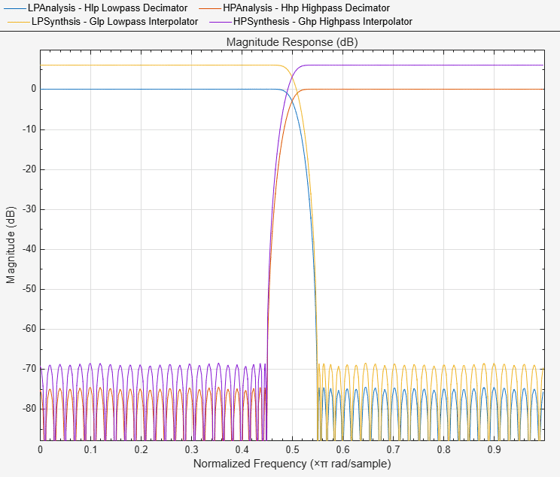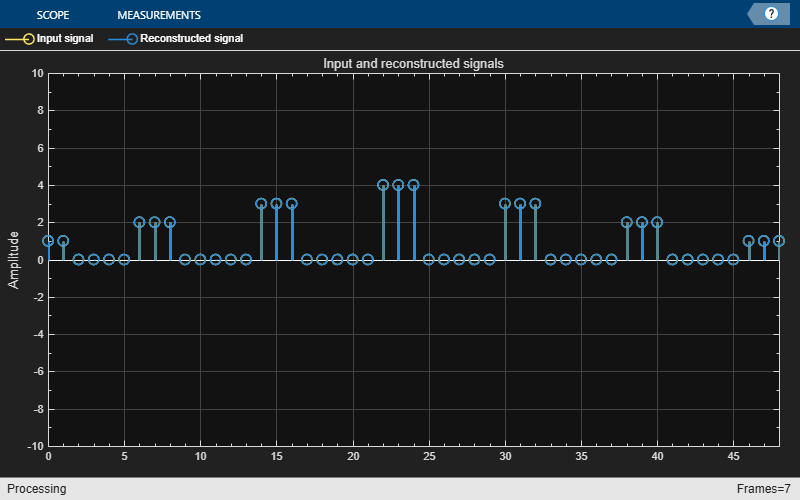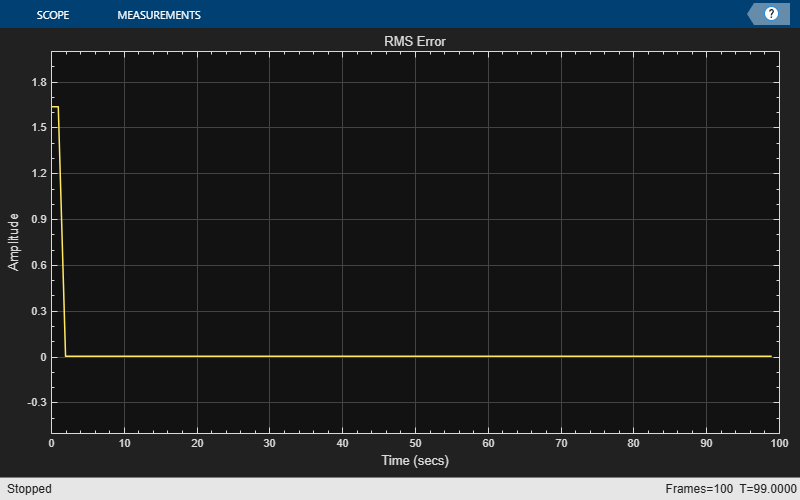### Perfect Reconstruction Output Analysis

We can see from the first two plots our perfect reconstruction two-channel filter bank completely reconstructed our original signal x[n]. The initial error is due to delay in the filters. The third plot shows that the cascade of subband filters do not modify the frequency characteristics of the signal.

### Application of Subband Filters - Audio Processing

Subband filters allow us to process the high frequencies in a signal in a way that is different from the way the low frequencies are processed. As an example, we will load an audio file and quantize its low frequencies with a wordlength that is higher than that of the high frequencies. The reconstruction then may not be perfect as above, but it still will be a reasonably accurate one.

Note: This requires a license for Fixed-Point Designer

First, create System object for loading and playing the audio file.

```audioInput = dsp.AudioFileReader; audioWriter = audioDeviceWriter('SampleRate',audioInput.SampleRate); ```

To have a measure of reference, we can play the original audio once

```while ~isDone(audioInput) input = audioInput(); % Load a frame audioWriter(input); % Play the frame end % Wait until audio is played to the end pause(10*audioInput.SamplesPerFrame/audioInput.SampleRate); reset(audioInput); % Reset to beginning of the file release(audioInput); % Close input file release(audioWriter); % Close audio output device ```

Next, we want to reset the subband filters from the above example of perfect reconstruction so that we can reuse them. Release method is called on plots to be able to change certain properties.

```hide(errorPlot) hide(tfplot) reset(analysisFilter); release(analysisFilter); % Release to change input data size reset(synthFilter); release(synthFilter); % Release to change input data size % Plot for error errorPlot.SampleRate = audioInput.SampleRate/audioInput.SamplesPerFrame; errorPlot.TimeSpan = 5.5; clear sigcompare % Do not need a plot to compare signals reset(tfestimate); % Transfer function estimate release(tfestimate); release(tfplot); % Plot for transfer function estimate tfplot.YLimits = [-20, 60]; tfplot.XOffset = -audioInput.SampleRate/2; tfplot.SampleIncrement = ... audioInput.SampleRate/audioInput.SamplesPerFrame; ```

The simulation loop is very similar to the one for the perfect reconstruction example in the beginning. The changes here are:

1. Quantization is performed for the low frequency component to have 8 bits and the high frequency to have 4 bits of wordlength.

2. The reconstructed audio is played back to let the user hear it and notice any changes from the input audio file. Note that the quantized subbands are saved in `double` container because the `dsp.SubbandSynthesisFilter` object needs its inputs to be of the same numerictype.

```show(tfplot) show(errorPlot) while ~isDone(audioInput) input = audioInput(); % Load a frame of audio [hi, lo] = analysisFilter(input); % Analysis QuantizedHi = double(fi(hi, 1, 4, 9)); % Quantize to 4 bits QuantizedLo = double(fi(lo, 1, 8, 8)); % Quantize to 8 bits reconstructed = synthFilter(QuantizedHi, QuantizedLo); % Synthesis % Plot error between signals err = rms(input(2:end) - reconstructed(1:end-1)); errorPlot(err); % Play the reconstructed audio frame audioWriter(reconstructed); % Estimate transfer function of cascade Txy = tfestimate(input(2:end), reconstructed(1:end-1)); tfplot(20*log10(abs(Txy))); end release(errorPlot); % Wait until audio is played to the end pause(10*audioInput.SamplesPerFrame/audioInput.SampleRate); release(audioWriter); % Close audio output device reset(audioInput); % Reset to beginning of the file release(audioInput); % Close input file ```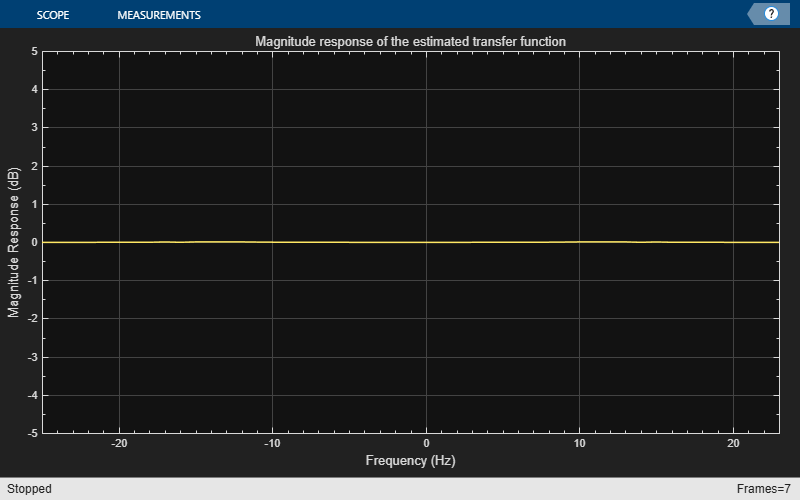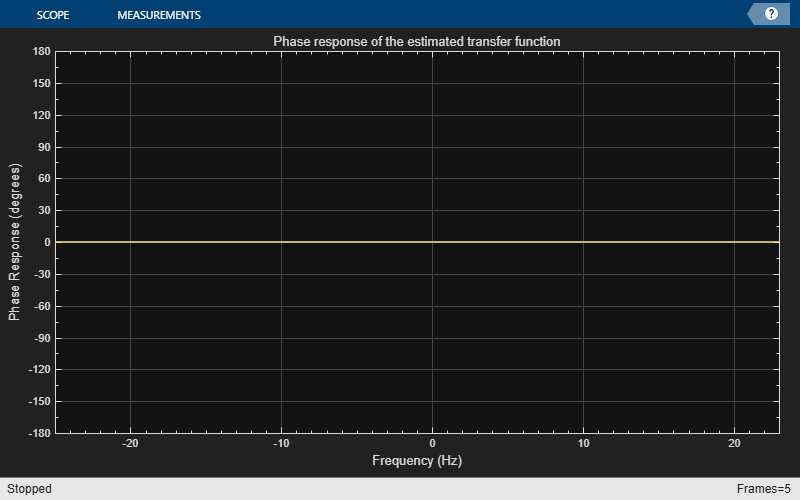As the error plot shows, the reconstruction is not perfect because of the quantization. Also, unlike the previous case, the transfer function estimate of the complete system is also not 0dB. The gain can be seen to be different for low frequencies than for higher ones. However, on hearing the playback for reconstructed audio signal, you would have observed that the human ear is not too perceptible to the change in resolution, more so in the case of high frequencies where the wordlength was even lesser.

Watch now